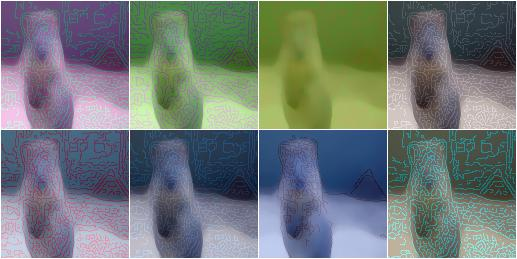# augmenters.edges¶

## Canny¶

Apply a canny edge detector to input images.

API link: `Canny`

Example. Create an augmenter that generates random blends between images and their canny edge representations:

```import imgaug.augmenters as iaa
aug = iaa.Canny()
```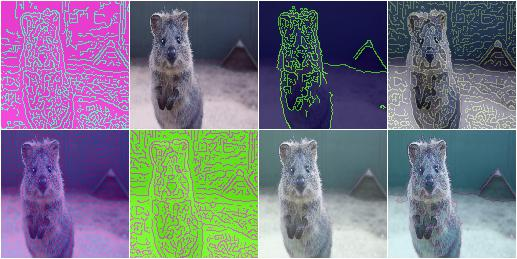Example. Create a canny edge augmenter that generates edge images with a blending factor of max `50%`, i.e. the original (non-edge) image is always at least partially visible:

```aug = iaa.Canny(alpha=(0.0, 0.5))
```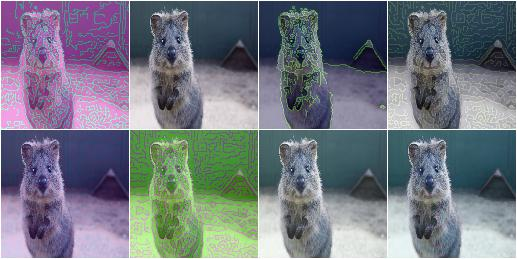Example. Same as in the previous example, but the edge image always uses the color white for edges and black for the background:

```aug = iaa.Canny(
alpha=(0.0, 0.5),
colorizer=iaa.RandomColorsBinaryImageColorizer(
color_true=255,
color_false=0
)
)
```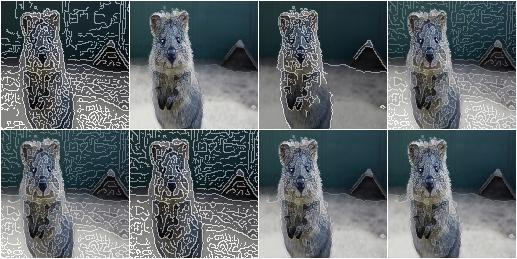Example. Create a canny edge augmenter that initially preprocesses images using a sobel filter with kernel size of either `3x3` or `13x13` and alpha-blends with result using a strength of `50%` (both images equally visible) to `100%` (only edge image visible).

```aug = iaa.Canny(alpha=(0.5, 1.0), sobel_kernel_size=[3, 7])
```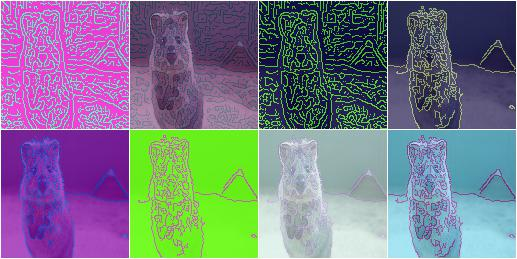Example. Create an augmenter that blends a canny edge image with a median-blurred version of the input image. The median blur uses a fixed kernel size of `13x13` pixels.

```aug = iaa.Alpha(
(0.0, 1.0),
iaa.Canny(alpha=1),
iaa.MedianBlur(13)
)
```